Advanced Database Management System - Tutorials and Notes: Language model in natural language processing

## Language Model in Natural Language Processing

A statistical language model is a probability distribution over sequences of strings/words, and assigns a probability to every string in the language.

Language models are based on a probabilistic description of language phenomena. Probabilities are used to pick the most frequent of several alternatives. Hence, probabilistic langue models used in various NLP applications including spelling correction, speech recognition, syntactic analysis, text summarization, word prediction, etc. to predict the most probable word sequence (or sentence). You would probably understand on "how probability contributes on some of these applications?" from the example below;

Example:

 NLP Application Probability comparison between correct and wrong sequences of words Spell correction ➔ P(I love flowers) > P(I lvoe flowers) Speech recognition ➔ P(I saw a man) > P(eyes awe a man) Sentence formation ➔ P(I am doing law) > P(am law doing I) Word prediction ➔ P(Please turn the lights on) > P(Please turn the lights right)

How to compute the probability of a sentence?
A sentence (W) is a sequence of words (w1, w2, …, wn) and the probability of the same can be calculated as follows;

P(W) = P(w1, w2, …, wn)
Also, the probability of an upcoming word can be calculated of a given word sequence;
P(wn | w1, w2, …, wn-1)

The model that calculates either P(W) or P(w1, w2, …, wn) is called the language model.

How to calculate P(w1, w2, …, wn)?
P(w1, w2, …, wn) is a joint probability. Let us calculate the joint probability P(A, B) for two events A and B.
The joint probability can be calculated using the conditional probability as follows;
Conditional probability,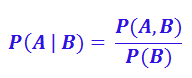which can be rewritten as,

P(A, B) = P(A | B) * P(B)

By Bayes’ theorem to manipulate conditional probability, we can further expand the joint probability equation as follows;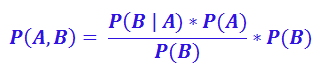P(A, B) = P(A) * P(B | A)

Chain rule of probability:
The above derived joint probability can be extended to more variables as follows;

P(A, B, C, D) = P(A) * P(B | A) * P(C | A, B) * P(D | A, B, C)

This is referred as chain rule. This can be generalized and used to calculate the joint probability of our word sequence P(w1, w2, …, wn) as follows;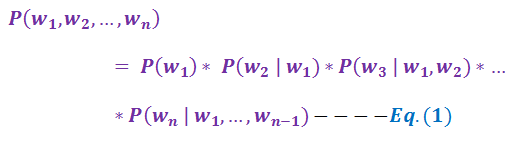The chain rule can be used to find the probability of given sequence of words. For example, probability of the sentence (word sequence) “the prime minister of our country” can be calculated as follows;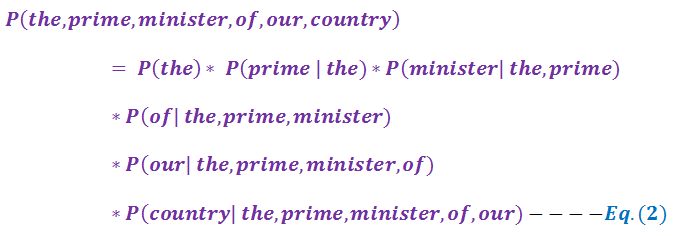Now, if you like to compute the probability of the word sequence W, we need to calculate each component in it. For instance, for the example given in Equation 2, we need to measure the component P(our|the prime minister of). One way to measure this is relative frequency count which is as follows;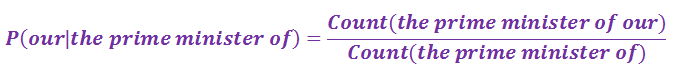This can be read as, "out of the number of times we saw ‘the prime minister of’ in a corpus, how many times was it followed by the word ‘our’".

Due to sparseness of data and the length of the given test data (sentence), one or more of the components in the above equation may 0 which leads the result to 0. How do we handle this problem?

Markov assumption:
The probability of a word depends only on the previous word or previous couple of words. According to this assumption, we do not need to look too far in the history to predict the future. Instead, we can approximate the history by just the last few words. As per the Markov assumption, one of the components in the Equation 2 of our example can be modified as follows;

P(our | the prime minister of) ≈ P(our | of)
OR
P(our | the prime minister of) ≈ P(our | minister of)

Here, the first one is bi-gram model, and the second one is tri-gram model.
As per the bigram model, we are making the following approximation;

P(wn | w1, w2, …, wn-1) ≈ P(wn | wn-1)

As per the trigram model, we are making the following approximation;

P(wn | w1, w2, …, wn-1) ≈ P(wn | wn-1, wn-1)

These models can be generalized as N-gram model which looks N-1 words from the history. The N-gram approximation to the conditional probability of the next words in a sequence is;

P(wn | w1, w2, …, wn-1) ≈ P(wn | wn-k, …, wn-1)

Here, k represents the particular model. For instance, k=1 refers to Unigram model, k=2 refers to Bigram model, k=3 refers to Trigram model, and so on.

----------------------------------------------------------------------------------------------------------------------------